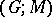# Transformation group

A permutation groupacting on a set. If, moreover,is endowed with a certain structure and if the elements ofpreserve this structure, then one appropriately says thatis a transformation group of this structure. The name of the transformation group reflects to a certain extent the name of the structure endowed on. E.g. ifis a vector space over a skew-field, then groups preserving this structure are called linear groups (cf. Linear group). Moreover, automorphism groups of modules over various rings are often called linear groups. In particular, ifis a free finite-dimensional module over the ringof integers, one speaks of crystallographic groups (cf. Crystallographic group). Ifis a topological space andconsists of the automorphisms of, one speaks of continuous transformation groups. Ifis a field andis a finite automorphism group of, thenis the Galois group of the extension, whereis the subfield of elements that are fixed under the action by elements of. One can also consider situations in whichandare endowed with structures of the same type and where the action ofonis a morphism in the corresponding category. E.g. ifis a topological group continuously acting on a topological space, one speaks of a topological transformation group (cf. Topological group); Lie transformation groups and algebraic transformation groups are defined similarly (cf. Lie transformation group; Algebraic group of transformations).